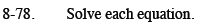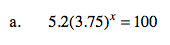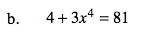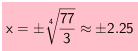### Home > CCA2 > Chapter 8 > Lesson 8.2.1 > Problem8-78

8-78.
1. Solve each equation. Homework Help ✎

1. 5.2(3.75)x = 100

2. 4 + 3x4 = 81Divide by 5.2 and then use logarithms.

x ≈ 2.24Isolate x4 then use roots or fractional exponents.